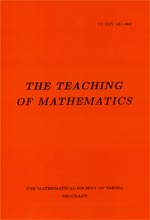﻿ The Teaching of Mathematics - Instructions for Authors ﻿
 THE TEACHING OF MATHEMATICSOn some properties of triangle $OIG$ Yu. N. Maltsev and A. S. Monastyreva AbstractLet $O$ be the circumcenter of a triangle $ABC$, $I$ the incenter and $G$ the centroid of $ABC$. In this paper, we study properties of the triangle $OIG$.Keywords: Centroid; circumcenter; incenter. Pages:  102$-$108 Volume  XXIII ,  Issue  2 ,  2020

﻿